Thermodynamic Studies of Trans-4-Hydroxy-L-Proline at Different Ionic Medium, Ionic Strength and Temperature | OMICS International
Journal of Bioprocessing & Biotechniques

Like us on:

All submissions of the EM system will be redirected to Online Manuscript Submission System. Authors are requested to submit articles directly to Online Manuscript Submission System of respective journal.

Thermodynamic Studies of Trans-4-Hydroxy-L-Proline at Different Ionic Medium, Ionic Strength and Temperature

 Emilia Furia*, Anna Napoli, Antonio Tagarelli and Giovanni Sindona Department of Chemistry and Chemical Technologies, University of Calabria, 87036 Arcavacata di Rende (CS), Italy Corresponding Author : Emilia Furia Department of Chemistry and Chemical Technologies University of Calabria 87036 Arcavacata di Rende (CS), Italy Tel: 0984-493328 Email: [email protected] Received November 29, 2013; Accepted January 24, 2014; Published January 31, 2014 Citation: Furia E, Napoli A, Tagarelli A, Sindona G (2014) Thermodynamic Studies of Trans-4-Hydroxy-L-Proline at Different Ionic Medium, Ionic Strength and Temperature. J Bioprocess Biotech 4:149. doi: 10.4172/2155-9821.1000149 Copyright: © 2014 Furia E, et al. This is an open-access article distributed under the terms of the Creative Commons Attribution License, which permits unrestricted use, distribution, and reproduction in any medium, provided the original author and source are credited.

Visit for more related articles at Journal of Bioprocessing & Biotechniques

Abstract

The aim of this work was to determine the solubility as well as the acidicconstants of trans-4-hydroxy-L-proline in 0.16 and 3.5 Mol.kg-1 NaClO4 and in 0.16 and 3.18 Mol.kg-1 NaCl at two different temperature, i.e. 298.15 and 310.15 K. trans-4-Hydroxy-L-proline, HL, is one of the most abundant amino acids present in collagen. As a major part of this protein, the measurement of hydroxyproline levels can be used as an indicator of collagen content. The protonation constants of ligand, K1 and K2, combined with the salting effects on the ligand in the ionic media were treated by the specific ion interaction theory, SIT, to give equilibrium constants at the infinite dilution reference state at 298.15 K and at 310.15 K, as well as specific interaction coefficients of the cation and anion of trans-4-hydroxy-L-proline with the media ions at the standard temperature, i.e. 298.15 K.

 Keywords Trans-4-Hydroxy-L-proline; Acidic constants; Constant ionic mediummethod; Infinite dilution reference state; SIT model Introduction In our on-going investigation of metals with biological ligands, we were interested in the metal–trans–4–hydroxy–L–proline complex formation equilibria. trans–4–Hydroxy–L–proline, HL (Figure 1), a constituent of several major structural proteins and other biodegradable synthetic products, is one of the main nonessential amino acid which contribute to the formation of collagen, the most abundant protein in humans as a primary constituent of bone, skin and connective tissues. Defects in collagen synthesis lead to easy bruising, internal bleeding, breakdown of connective tissue of the ligaments and tendons, and increased risk of blood vessel damage. Studies on the properties of trans–4–hydroxy–L–proline in aqueous solution are useful in understanding the details of the mechanism of its action in aqueous systems. Previous works [1,2] reported thermodynamic data about equilibrium constants of HL at 0.16 Mol.kg-1at two different temperature (i.e.298.15 and 310.15 K). By contrast, other available values  were obtained in non–aqueous solvents. In the present work the dependence of equilibrium constants of the ligand on ionic strength and media as well as on temperature was studied. It seems reasonable to predict different values of acidic constants at different ionic strength, medium and temperature. Thus, we have evaluated acidic constants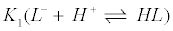and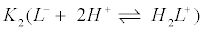of the lig and at two different ionic strength, I, in two distinct media, i.e.0.16 and 3.5 Mol.kg-1 NaClO4 and 0.16 and 3.18 Mol.kg-1 NaCl, as well as at the two different temperatures 298.15 and 310.15 K. The adoption of the constant ionic medium method, proposed by Biedermann and Sillén , was necessary in order to minimize activity coefficient variation in spite of the change of the reagent concentrations. By this approach, it was possible to replace in the calculations activities with concentrations and to minimize the liquid junction potential due to the hydrogen ion concentration. This was varied in order to determine acidic constants of ligand, taking into account that protonation takes place at different acidities. The potentiometric results and the solubility data obtained in the ionic media were processed by the specific ion interaction theory, SIT, [5,6] to yield acidic constants at the infinite dilution reference state and specific interaction coefficient of the cation and anion of HL with the media ions. Materials and Methods Apparatus and reagents The cell arrangement was as formerly described . The test solutions, stirred during titrations, were purified with a slow stream of nitrogen gas as reported in a previous work . Glass electrodes were the same used in preceding evaluations ; they attained a constant potential within few minutes after the addition of the reagents and remained unchanged for several hours to within ± 0.1 mV. The titrations were carried out as described in a previous paper . The electromotive force values were recorded with a precision of ± 10–5 V by an OPA 111 low–noise precision DIFET operational amplifier. The cell assembly was retained in a thermostat kept at (298.1 ± 0.1) and (310.1 ± 0.1) K. A perchloric acid and an hydrochloric acid stock solutions were prepared and standardized as described previously [9,11]. Sodium perchlorate stock solutions were prepared and standardized according to Biedermann . Sodium hydroxide titrant solutions were prepared and standardize as reported formerly . Purissimum grade (100 % Aldrich p.a.) trans–4–hydroxy–L–proline product was kept in a desiccator over silica gel and it was utilized without purification. All solutions were prepared with twice distilled water. Solubility measurements Solubility studies are of both theoretical and practical interest and they allow the determination of activity coefficients for nonelectrolyte solutes in aqueous solutions containing a large excess of salts [13-15]. The knowledge of the activity coefficients of neutral species is necessary when modeling the dependence of protonation constants on ionic strength according to the SIT equations [5,6]. Saturated HL were prepared as already described in a previous work . Solid HL was wrapped up in a highly retentive filter paper (Whatman 42) bag and then was kept in a glass cylinder containing pure water as well as sodium perchlorate and sodium chloride aqueous solutions at pre– established ionic strength values (0.16 and 3.5 Mol.kg-1, and 0.16 and 3.18 Mol.kg-1, respectively), under continuous stirring with a magnetic bar (Figure 2). The cylinder was then placed in a thermostatic water bath at (298.1 ± 0.1) and (310.1 ± 0.1) K and the ligand concentration was detected over time, until it reached a constant value, which usually occurred in about 3 up to 5 days. Finally, the absorption spectra in the UV region were recorded on a series of HL solutions. The absorbance, Aλ, may be expressed as equation 1: Aλ= ιε[HL]                                                            (1) Whereι is the optical path and ε is the molar absorptivity. To find suitable conditions for determining the solubility, S, of HL, Aλ were measured between 200 and 300 nm taking as a blank the ionic medium. Three replicates were run for each point. A typical spectrum of ligand recorded is reported in Figure 3. Absorption spectrum of HL shows one single intense band centered at 193.5 nm. The solubility, S, was deduced by interpolation on a calibration curve, based on standard solutions. The reproducibility of the solubility data was of 1%. Taking into account that S° is the solubility of the neutral species in the electrolyte solutions, the total solubility, S, of HL can be written as follows: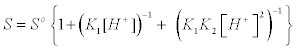(2) where the acidic constants of HL to the equilibria 3 and 4: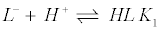(3)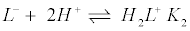(4) The solubility of the HL, valid in the molal concentration scale, is related to activity coefficient , γ, by the equation 5: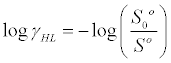(5) Where S0° is the solubility of HL at the infinite dilution reference state. The results are summarized in Table 1. Trans–4–Hydroxy–L–proline exhibits salting–in  behaviour, the solubilityincreasing monotonically with increasing ionic strength. Potentiometric measurements The protonation equilibria of trans–4–hydroxy–L–proline were investigated by potentiometric titrations with an alkaline glass electrode, GE, at 298.15 and at 310.15 K with cell (G) RE / Test Solution / GE (G) in which RE stands for the silver reference electrode = Ag/ AgCl/0.0105 Mol.kg-1 AgClO4, (I – 0.0105) molkg-1 NaClO4/IMol.kg-1 NaClO4, when NaClO4 was inert salt at two different ionic strength I (i.e. 0.16 and 3.5 Mol.kg-1) and = Ag / AgCl / IMol.kg-1NaCl saturated with AgCl / IMol.kg-1NaCl, when NaCl was inert salt at two different ionic strength I (i.e. 0.16 and 3.18 Mol.kg-1). Test solution had the general composition: CLMol.kg-1HL, CAMol.kg-1H+, CBMol.kg-1NaOH, (I – CA– CB) Mol.kg-1Na+, where CL were between (5 10-3 and 10 10-3) Mol.kg- 1 and I was 0.16 and 3.5 Mol.kg-1NaClO4,and 0.16 and 3.18 NaCl Mol.kg-1. The equilibrium constants at the infinite dilution reference state can be readily evaluated from results obtained in this ionic strength range according to the SIT . At a given I the electromotive force of cell (G) can be written, in mV, at the temperatures of 298.15 and 310.15 K as equations 6 and 7, respectively: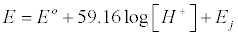(6)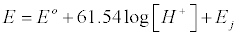(7) Where E° is constant in each series of measurements and Ej is the liquid junction potential  which is a linear function of [H+],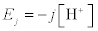. The j parameters, at the different ionic strengths, are known from a previous evaluation [9,18]. In each run E° values, constant within 0.1 mV, were calculated from measurements in solutions of (10-3 ≥ [H+] ≥110-4)mol kg-1 in the absence of HL. [H+] was decreased stepwise by coulometric generation of OH– ions with the circuit (C) – Pt / Test Solution / AE + (C) where AE, auxiliary electrode,=IMol.kg-1 inert salt/0.1 Mol. kg-1NaCl, (I–0.1) Mol.kg-1 Na+/Hg2Cl2/Hg. In the test solution of a given volume V dm3, CB=(μF 10-6/V) mol dm-3 where μF stands for the micro– faradays passed through the cell, according tothe assumption that at the cathode the only reactions that occur are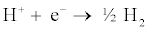and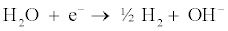After the introduction of a known amount of HL, dissolved in a known excess of CBMol.kg-1NaOH, the acidification was achieved, in the pH range from 12 to 2, by adding H+ standardized solutions, according to the equilibria reported in equations 3 and 4:The primary CL, CA, CB and [H+] data form the basis of the treatment to obtain the equilibrium constants. Results and Discussion The protonation constants of trans–4–hydroxy–L–proline, K1 and K2, were calculated from the data acquired by performing two titrations for each of the involved equilibria for the different ionic media and strengths and for the two different temperatures (298.15 and 310.15 K). In particular, a data set of 192 experimental points was used. An overview of the working conditions of all titrations carried out in NaClO4 and NaCl media is reported in Table 2 and Table 3, respectively. The experimental data (CL, CA, CB, [H+]) were processed by numerical procedures. For the numerical treatment we employed the least–squares computer program Superquad  to seek the minimum of the function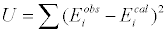(8) Where Eobs = Eo + 59.16log[H+ ] and Eobs = Eo + 61.54log[H+ ] at 298.15 and 310.15 K, respectively, while Ecal is a value calculated for a given set of parameters. In the numerical treatment the ion product of water has been taken from the literature  for different temperature and different ionic strength and media. Results are given in Table 4 and the uncertainties on equilibrium constants represent 3σ. As it can be seen in Table 4 the value of the acidic constants increases as temperature increases, thus supporting that reactions are thermodynamically favored. Dependence on ionic medium, ionic strength and temperature by SIT approach The acidic constants at the infinite dilution reference state, °K1 and °K2, of HL were determined by the numerical values reported in Table 4, by assuming the validity of the SIT . According to this theory, constants and other quantities in the following treatment are expressed on the molal scale by using the conversion factors from Grenthe et al. . The activity coefficient, Zi, of the species i with charge zi can be expressed inaqueous solution as stated by the SIT: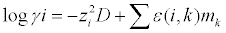(9) Where D is the Debye-Hückel term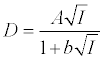(10) Where the b value is arbitrarily chosen, generally 1≤b≤1.5. In equation 9 ε is the specific ion interaction coefficient of i with species k of molality mk. The SIT model is based on the assumption that interaction coefficients of ions with the same charge type are nearly zero. Interaction coefficients are the result of short range forces and depend on the ionic strength but their variation in the range 0.5 ≤ I ≤ 3.5molal is sufficiently low that they may be assumed as constants. Parameters A and b in equation 10 can be taken from the literature. In particular, b = 1.5 [22,23], while A varies with temperature [24,25]. In the range 273≤T≤348 K AT can defined as follows :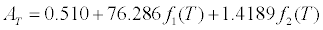(11) Where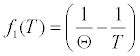(12)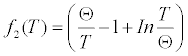(13) and is the standard temperature, i.e. 298.15 K. According to the specific interaction theory [5,6] the protonation constants of trans–4– hydroxy–L–proline, K1 and K2, combined with the salting effects of inert salts (i.e. NaClO4 and NaCl) on HL deduced from the solubility determinations, were processed to give equilibrium constants at the infinite dilution reference state, 1 °K and 2 °K . The effect of the ionic media at different ionic strength (i.e.0.16 and 3.5 Mol.kg-1 NaClO4, and in 0.16 and 3.18 Mol.kg-1NaCl) on the various equilibrium constants (according to equilibria 3 and 4) determined in this work can be expressed as: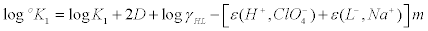(14)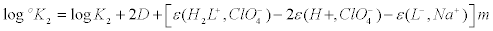(15) when inert salt is NaClO4, and as: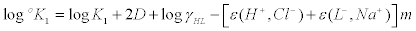(16)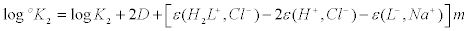(17) When inert salt is NaCl. The activity coefficients of HL, log HL γ , were deduced bysolubility measurements in the electrolyte solutions, and from Ciavatta  at 298.15 K ε (H+, ClO4 –) = 0.14 and ε (H+, Cl–) = 0.12. Hence, plots on the known terms of equations 14-17 as a function of I result in straight lines in which the intercept correspond to the constants at zero ionic strength and the slope are the interaction coefficients, ε (i, k), between HL and the counter ions of the ionic media (Table 5). The interaction coefficients between Na+ ion and the anion of the acid as well as between ClO4- ion and H2L+ agree with those expected for small ion . As concerning ε (H2L+, Cl-) the value obtained is negligible. To calculate acidic constants of HL at the infinite dilution reference state at the temperature of 310.15 K, it was necessary to evaluate D, which is dependent on ionic strength I as well as on temperature by parameter AT (equation 11). Similarly to what has been done at 298.15 K, it was possible to calculate °K1 and °K2 at 310.15 K, by assuming the validity of the SIT and taking into account equilibria 3 and 4. However in this case it was possible to deduce just the algebraic sum of interaction coefficients ε (i,k) since ε (H+, ClO4 –) and ε (H+, Cl–) were unknown at 310.15 K. Results of extrapolation are reported in Table 6. The trend of two acidic constants at two different temperatures was, accordingly, matched at the two selected ionic media (Figure 4). A common behaviour was observed, since acidic constants increase as temperature increases. By contrast, a distinct effect of the ionic medium was clearly observed, whereby K1 decreases with different inert salt, while K2 results unaffected by the ionic medium. Conclusions The solubility and the acidic constants of trans–4–hydroxy–L– proline have been determined, at 298.15 and 310.15 K, in 0.16 and 3.5 Mol.kg-1 NaClO4 and in 0.16 and 3.18 mol kg-1 NaCl solutions and at the infinite dilution reference state. Results have been used to evaluate the salting effect of NaClO4 as well as of NaCl on the neutral molecule and interaction coefficients ε (i, k). The acidic constants obtained in this work and those taken from literature [1,2] are collected in Table 7 for a comparison. The reactions are thermodynamically favored since acidic constants increase as temperature increases. The agreement between the data sets is just satisfactory, especially as concerning log K2 by Makaret al.  at 310.15 K. The available values from other authors  were obtained in non–aqueous solvents, for this reason it was not possible to make any comparison. The results obtained in this work can be used for further studies regarding complex formation equilibria between HL and metal cations. References Zielinski S, Lomozik L, Wojciechowska A (1981) Potentiometric studies on the complex formation of lanthanides with proline and hydroxyproline. Monatsh Chem 112: 1245-1252. Makar GKR, Touche MLD, Williams DR (1976) Thermodynamic considerations in co-ordination. Part XXIII.1 Formation constants for complexes of protons, zinc(II), and acid anions and their use in computer evaluation of a better zinc therapeutical. J Chem Soc Dalton Trans 11: 1016-1019. Pettit GL (2006) IUPAC: Stability Constant Data Base. Tools of the Trade. Biedermann G, Sillén LG (1953) Studies on the hydrolysis of metal ions. IV. Liquid junction potentials and constancy of activity factors in NaClO4–HClO4 ionic medium. Ark Kem 5: 425-440. Ciavatta L (1980) The specific interaction theory in evaluating ionic equilibria. Ann Chim 70: 551-567. Ciavatta L (1990) The specific interaction theory in equilibrium analysis. Some empirical rules for estimating interaction coefficients of metal ion complexes. Ann Chim 80: 255-263. Furia E, Porto R (2008) 2–Hydroxybenzamide as ligand. Complex formation with dioxouranium(VI), aluminium(III), neodymium(III) and nickel(II) ions. J Chem Eng Data 53: 2739-2745. Furia E, Porto R (2004) The hydrogen salicylate ion as ligand. Complex formation equilibria with dioxouranium (VI), neodymium (III) and lead (II). Ann Chim 94: 795-804. Furia E, Porto R (2002) The effect of ionic strength on the complexation of copper (II) with salicylate ion. Ann Chim 92: 521-530. Furia E, Sindona G (2010) Complexation of L-cystine with metal cations. J Chem Eng Data 55: 2985-2989. Porto R, De Tommaso G, Furia E (2005) The second acidic constant of salicylic acid. Ann Chim 95: 551-558. Biedermann G (1964) Study on the hydrolysis equilibria of cations by emf methods. Ft. Belvoir Defense Technical Information Center. Long FA, McDevit WF (1952) Activity coefficients of nonelectrolyte solutes in aqueous salt solution. Chem Rev 51: 119-169. Randal M, Failey CF (1927) The activity coefficient of the undissociated part of weak electrolytes. Chem Rev 4: 291-318. Setschenow JZ (1889) Über die konstitution der salzlosungenauf grund ihres verhaltens zu kohlensaure. Z Phys Chem 4: 117-125. Furia E, Nardi M, Sindona G (2010) Standard potential and acidic constants of Oleuropein. J Chem Eng Data 55: 2824-2828. Osol A, Kilkpatrick M (1933) The “salting-out” and “salting-in” of weak acids. I. The activity coefficients of the molecules of ortho, meta and para chlorobenzoic acids in aqueous salt solutions. J Am Chem Soc 55: 4430-4440. Bottari E, Porto R (1986) Protolytic equilibria in aqueous sodium deoxycholate solutions. Monatsh Chem 117: 589-597. Gans P, Sabatini A, Vacca A (1985) SUPERQUAD: an improved general program for computation of formation constants from potentiometric data. J Chem Soc Dalton Trans 6: 1195-1200. Baes CF, Mesmer RE (1976) The Hydrolysis of Cations. A Wiley– Interscience Publication, New York. Grenthe I, Fuger J, Konigs RJM, Lemire RJ, Muller AB, et al., (2004) Chemical thermodynamics of Uranium. Nuclear Energy Agency, France. Bretti C, Foti C, Sammartano S (2004) A new approach in the use of SIT in determining the dependence on ionic strength of activity coefficients. Application to some chloride salts of interest in the speciation of natural fluids. Chem Spec Bioavailab 16: 105-110. Scatchard G (1976) Equilibrium in solution: surface and colloid chemistry. Harvard University Press, Cambridge, Massachusetts. Robinson RA, Stokes RH (1959) Electrolyte solutions. Butterworths scientific publications, London. Helgenson HC, Kirkham DH, Flowers GC (1981) Theoretical prediction of the thermodynamic behavior of aqueous electrolytes at high pressures and temperatures: IV. Calculation of activity coefficients, osmotic coefficients, and apparent molal and standard and relative partial molal properties to 600°C and 5kb. Am J Sci 281: 1249-1516.

Tables and Figures at a glanceTable 1 Table 2 Table 3 Table 4Table 5 Table 6 Table 7

Figures at a glance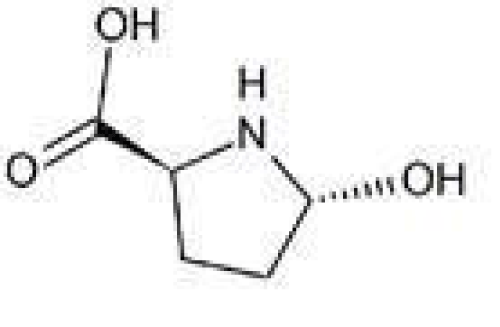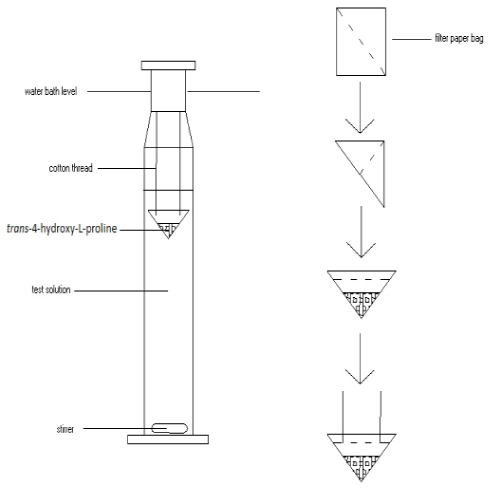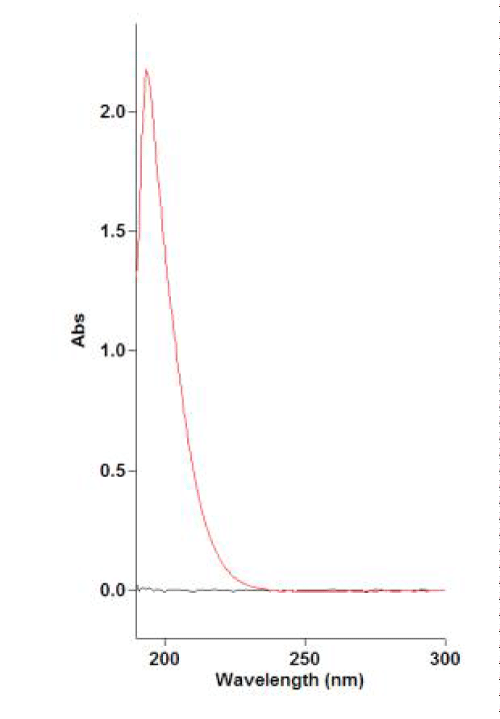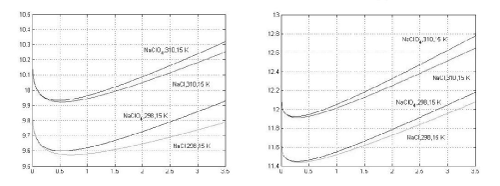Figure 1 Figure 2 Figure 3 Figure 4
Select your language of interest to view the total content in your interested language

Article Usage

• Total views: 11818
• [From(publication date):
April-2014 - Jun 18, 2019]
• Breakdown by view type
• HTML page views : 8016Can't read the image? click here to refresh